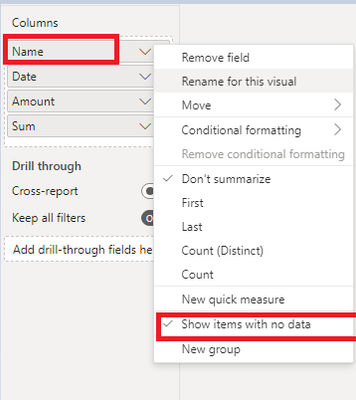cancel
Showing results for
Did you mean:Helper IV

## DAX Sum only if total per month is higher then X

Hi all,

My data looks like this

 Name date Amount Joe 1-1-2023 10 Joe 15-1-2023 20 Jane 2-1-2023 10 Jane 20-1-2023 10 Joe 1-2-2023 15 Jane 5-2-2023 30 Jane 10-2-2023 10

On my report page I have a filter month.

I want a measure that returns the sum of Amount only if the total of that month for that person is greater then 25.

For example when i Select Januari the result shoud be

Joe = 30
Jane = Blank

In februari

Joe = Blank

Jane = 40

Can you help me achieve this?

1 ACCEPTED SOLUTIONCommunity Support

You can create a measure,  the code is as follows:

``````Sum = var a= SUMX(FILTER(ALL('Table'),'Table'[Name]=SELECTEDVALUE('Table'[Name])&&MONTH('Table'[Date])=MONTH(SELECTEDVALUE('Table'[Date]))),[Amount])

return IF(ISFILTERED('Table'[Date].[Month]),IF(a>25,a,BLANK()),SUM('Table'[Amount]))``````

Then click the ‘Name’ field in the visual, set that it can be displayed with no dataOutputBest Regards,

Yolo Zhu

If this post helps, then please consider Accept it as the solution to help the other members find it more quickly.Community Support

You can create a measure,  the code is as follows:

``````Sum = var a= SUMX(FILTER(ALL('Table'),'Table'[Name]=SELECTEDVALUE('Table'[Name])&&MONTH('Table'[Date])=MONTH(SELECTEDVALUE('Table'[Date]))),[Amount])

return IF(ISFILTERED('Table'[Date].[Month]),IF(a>25,a,BLANK()),SUM('Table'[Amount]))``````

Then click the ‘Name’ field in the visual, set that it can be displayed with no dataOutputBest Regards,

Yolo Zhu

If this post helps, then please consider Accept it as the solution to help the other members find it more quickly.